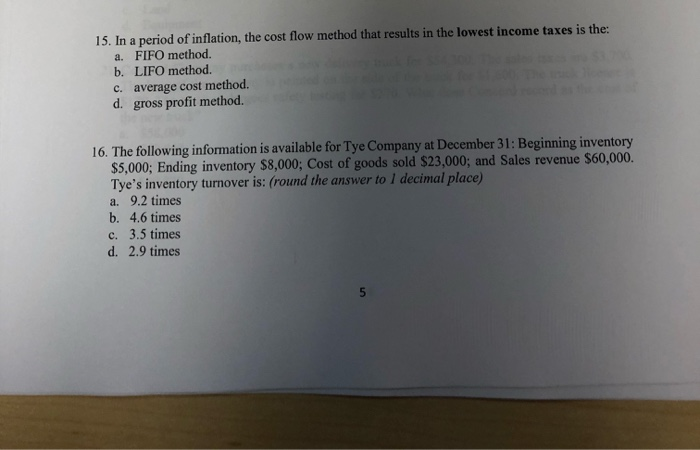# Question Solved1 Answer15. In a period of inflation, the cost flow method that results in the lowest income taxes is the: a. FIFO method. b. LIFO method. c. average cost method. d. gross profit method. 16. The following information is available for Tye Company at December 31: Beginning inventory \$5,000; Ending inventory \$8,000: Cost of goods sold \$23,000; and Sales revenue 15. In a period of inflation, the cost flow method that results in the lowest income taxes is the: a. FIFO method. b. LIFO method. c. average cost method. d. gross profit method. 16. The following information is available for Tye Company at December 31: Beginning inventory \$5,000; Ending inventory \$8,000: Cost of goods sold \$23,000; and Sales revenue \$60,000. Tye's inventory turnover is: (round the answer to I decimal place a. 9.2 times b. 4.6 times c. 3.5 times d. 2.9 timesTranscribed Image Text: 15. In a period of inflation, the cost flow method that results in the lowest income taxes is the: a. FIFO method. b. LIFO method. c. average cost method. d. gross profit method. 16. The following information is available for Tye Company at December 31: Beginning inventory \$5,000; Ending inventory \$8,000: Cost of goods sold \$23,000; and Sales revenue \$60,000. Tye's inventory turnover is: (round the answer to I decimal place a. 9.2 times b. 4.6 times c. 3.5 times d. 2.9 times
More
Transcribed Image Text: 15. In a period of inflation, the cost flow method that results in the lowest income taxes is the: a. FIFO method. b. LIFO method. c. average cost method. d. gross profit method. 16. The following information is available for Tye Company at December 31: Beginning inventory \$5,000; Ending inventory \$8,000: Cost of goods sold \$23,000; and Sales revenue \$60,000. Tye's inventory turnover is: (round the answer to I decimal place a. 9.2 times b. 4.6 times c. 3.5 times d. 2.9 times